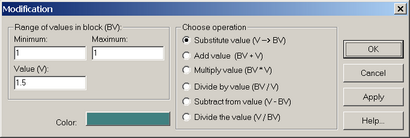﻿ Numerical modeling (ANSRADIAL) > Model editing > Graphic editor > Modify values

Modify values

The "Modification" dialog box allows modifying or replacing values on the grid and performing mathematical operations with a selected range of values.- call button in the graphic editor window."Modification" dialog box.

Explanatory table

 "Range of values in blocks (BV)" frame Specify the range of parameter values to be substituted. Modifications will take change in the blocks, in which the values fall into the specified range "Minimum" text box Minimum parameter value to be substituted "Maximum" text box Maximum parameter value to be substituted "Value (V)" text box The value with which mathematical operations are performed "Choose operation" frame Choose mathematical operation to be performed to substitute parameters from the selected range of values "Substitutes value (V -> BV)" option Substitute the value in the block (BV) by the specified value (V) "Adds value (BV + V)" option Add the specified value (V) to the value in the block (BV) "Multiplies value (BV * V)" option Multiply the value in block (BV) by the specified value (V) "Divides by value (BV / V)" option Divide the value in block (BV) by the specified value (V) "Subtracts from value (V - BV)" option Subtract the block value (BV) from the specified value (V) "Divides the value (V /BV)" option Divide the specified value (V) by the value in block (BV) "Color" button Selects color for a specified range selection

BV - parameter value in block.

V - value with which mathematical operations are performed.